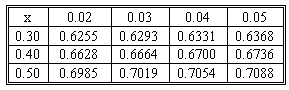### CFA Practice Question

There are 227 practice questions for this topic.

### CFA Practice Question

Consider a stock that trades for \$75. A call on this stock has an exercise price of \$70 and it expires in 150 days. If the continuously compounding interest rate is 7% and the standard deviation for the stock's return is 0.35, compute the price of the call option according to the Black-Scholes-Merton model.

Below is the relevant part of the cumulative probabilities table for a standard normal distribution.d1 = {ln(75/70) + [0.07 + (0.352/2)] x (150/365)} / (0.35 (150/365)1/2) = 0.5479
d2 = 0.5479 - 0.35 x (150/365)1/2 = 0.3235
N(d1) = N(0.55) = 0.7088
N(d2) = N(0.32) = 0.6255
c = \$75 x 0.7088 - \$70 x e -0.07 x (150/365) x 0.6255 = \$10.62